# Azure ML Titanic Example – Part 2 – Build Model

In the previous post we showed how to set up our data so it is ready for building our Azure ML model. Next, we will build our model. We will be trying to determine whether a passenger “survived” or not.

We have a field in our data for Survived, so we will set this as a label in Azure ML. To do this, we will drag across a new Edit Metadata, connect it to our previous  and select “Survived”: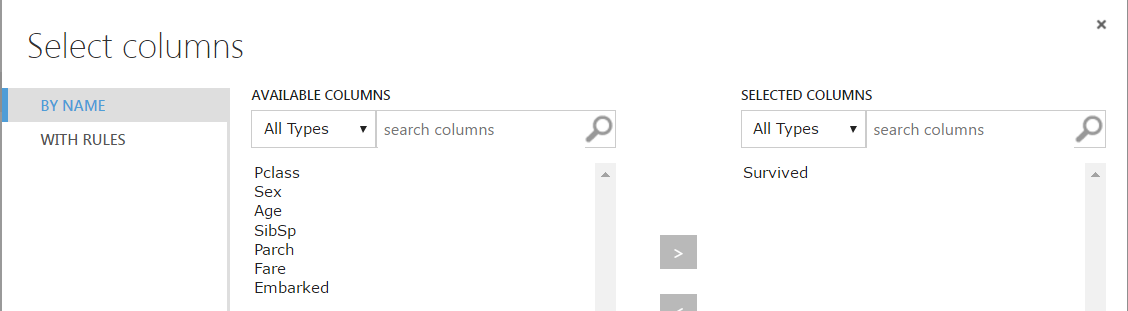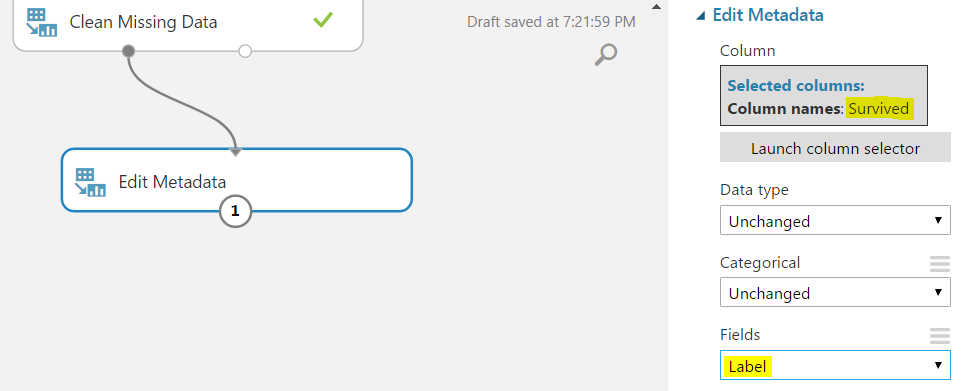Rerun the model. Survived is now a categorical label: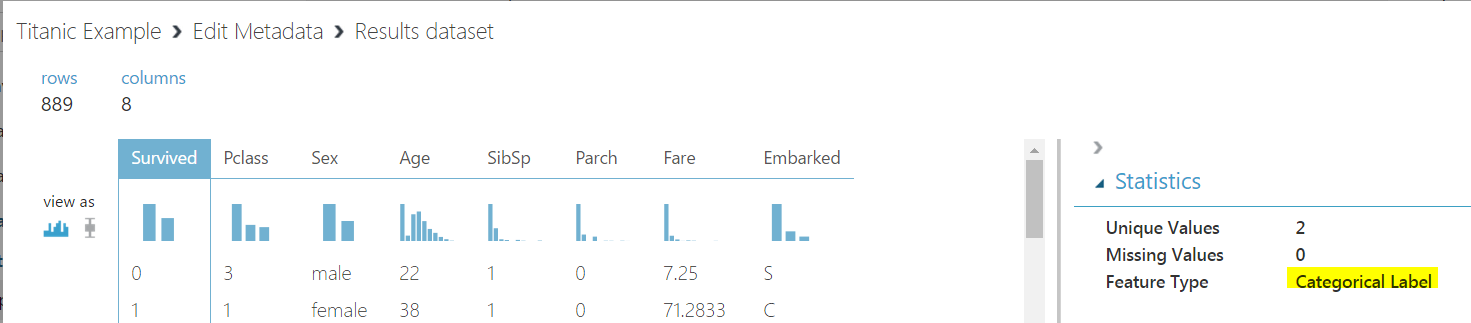Now we can split the data. Drag across Split: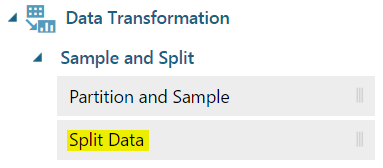We will split the data so that 70% (0.7) goes to the left and 30% to the right. The split will be random: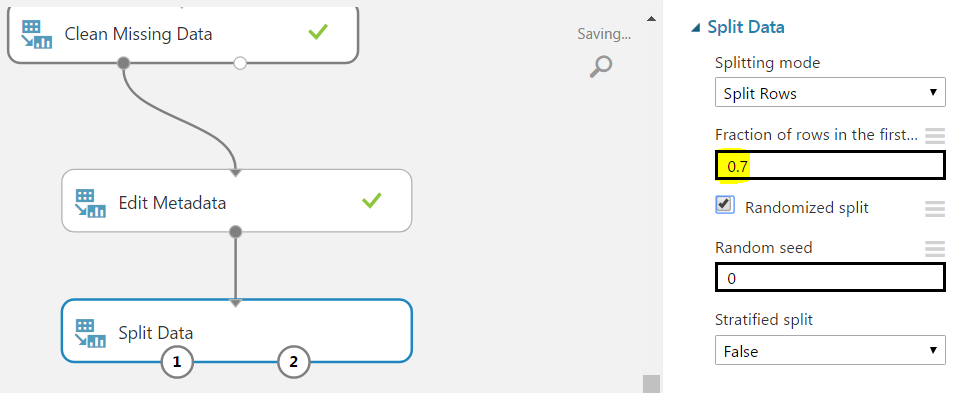Now we can determine what type of algorithm we will use on the data. We are trying to determine survived vs not survived, so this is a 2 class classification. To find the models, go to Machine Learning to see the 2-class algorithms available: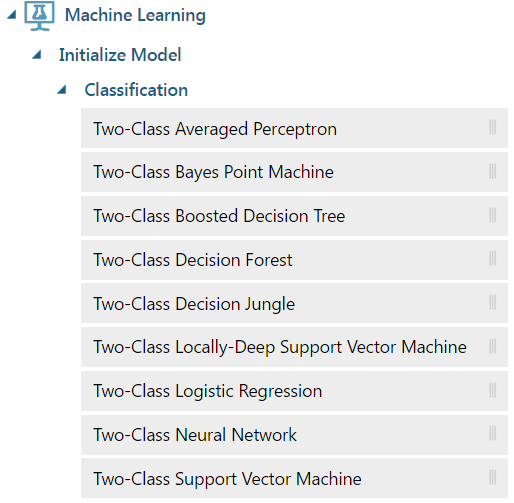We will try out a Two-Class Decision Forest. Drag this onto the canvas.

Now, we will need to also train, score and evaluate the model. Drag these onto the canvas as well.

The model should now look something like this: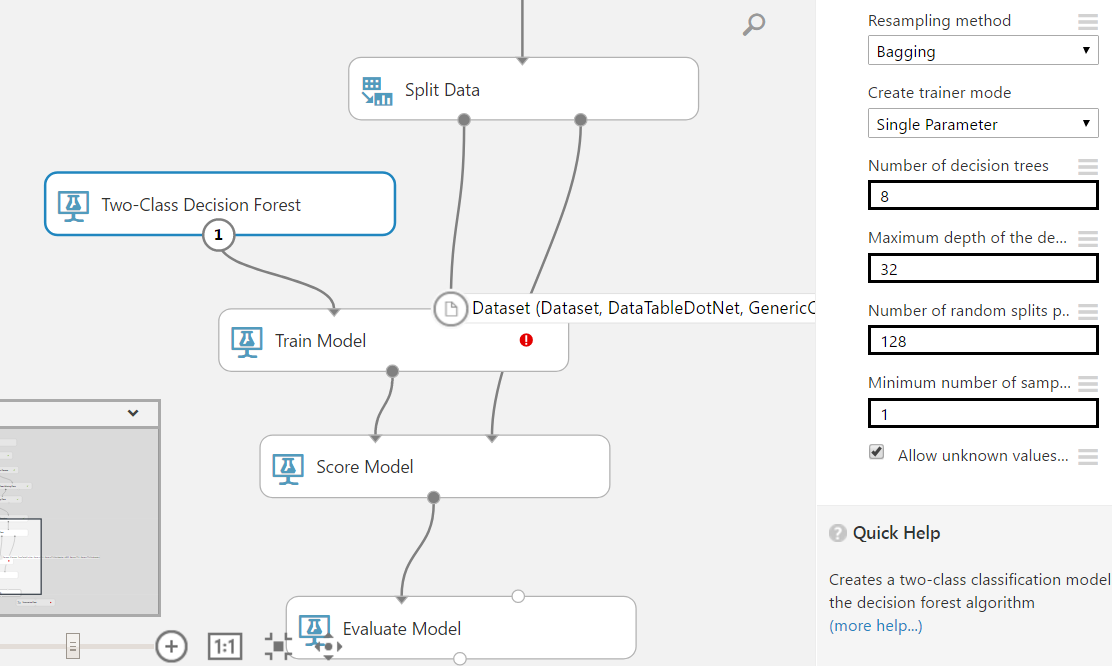Now we need to set the response of the Score Model. Select the model and chose the column “Survived”: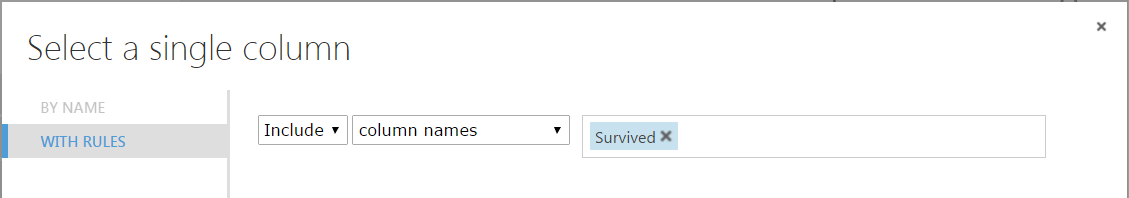We can now run our model to see how it performed. Click Run.

Now, right click on the Evaluation Model and Visualize.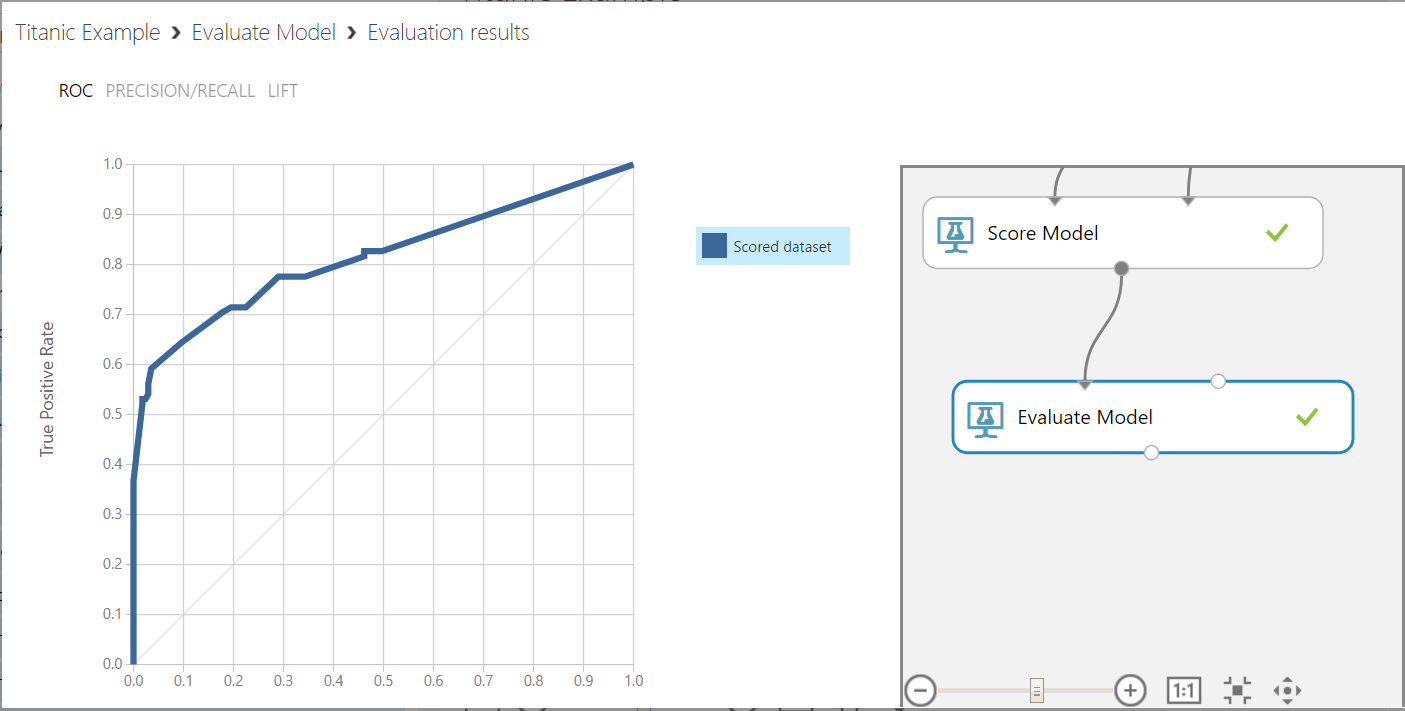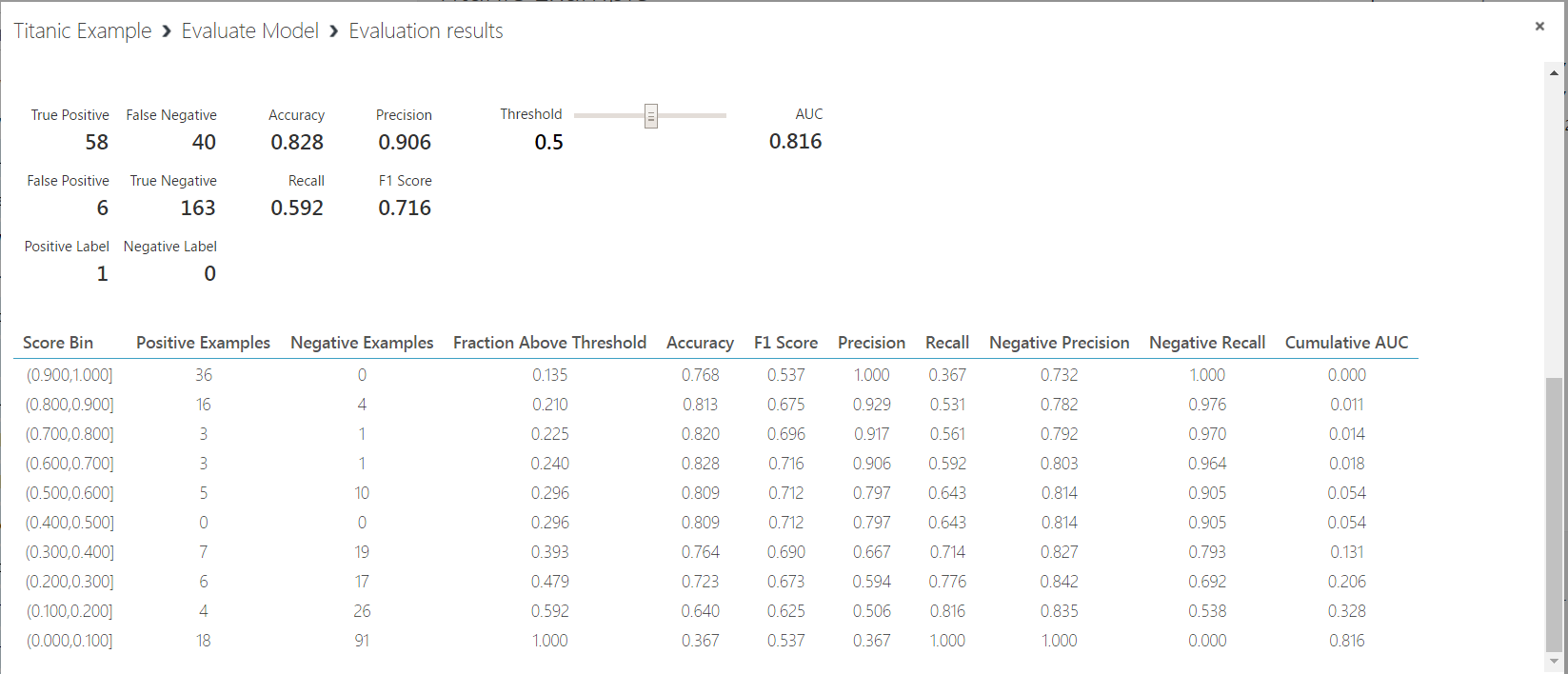We can see the model has a high precision (0.906) and accuracy (0.828). However, our recall value is low (0.592). This is the sensitivity, or true positive rate of our model.

Also, the F1 score is 0.716. This is the weighted average of Precision and Recall, or 2*(Recall * Precision)/(Recall + Precision).

We can then test different algorithms and evaulate them to find the best performing model. We can do this by adding multiple algorithms and comparing them against each other, or by swapping the algorithm used and checking its results. Here, we have are using the Two-Class Logical Regression algorithm: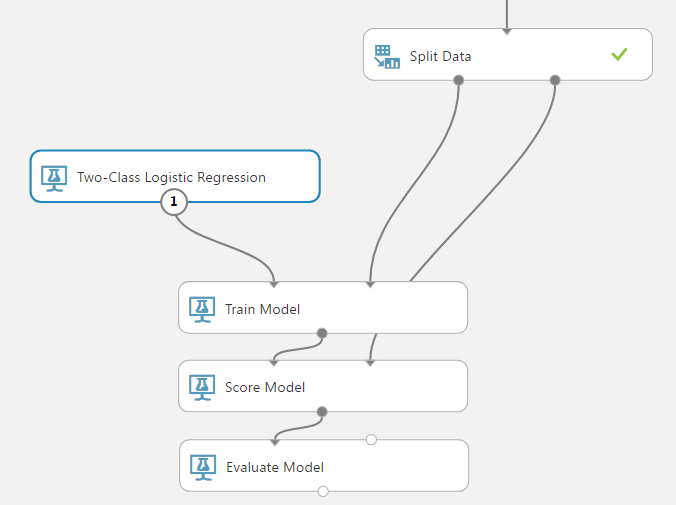Running this, we get the results: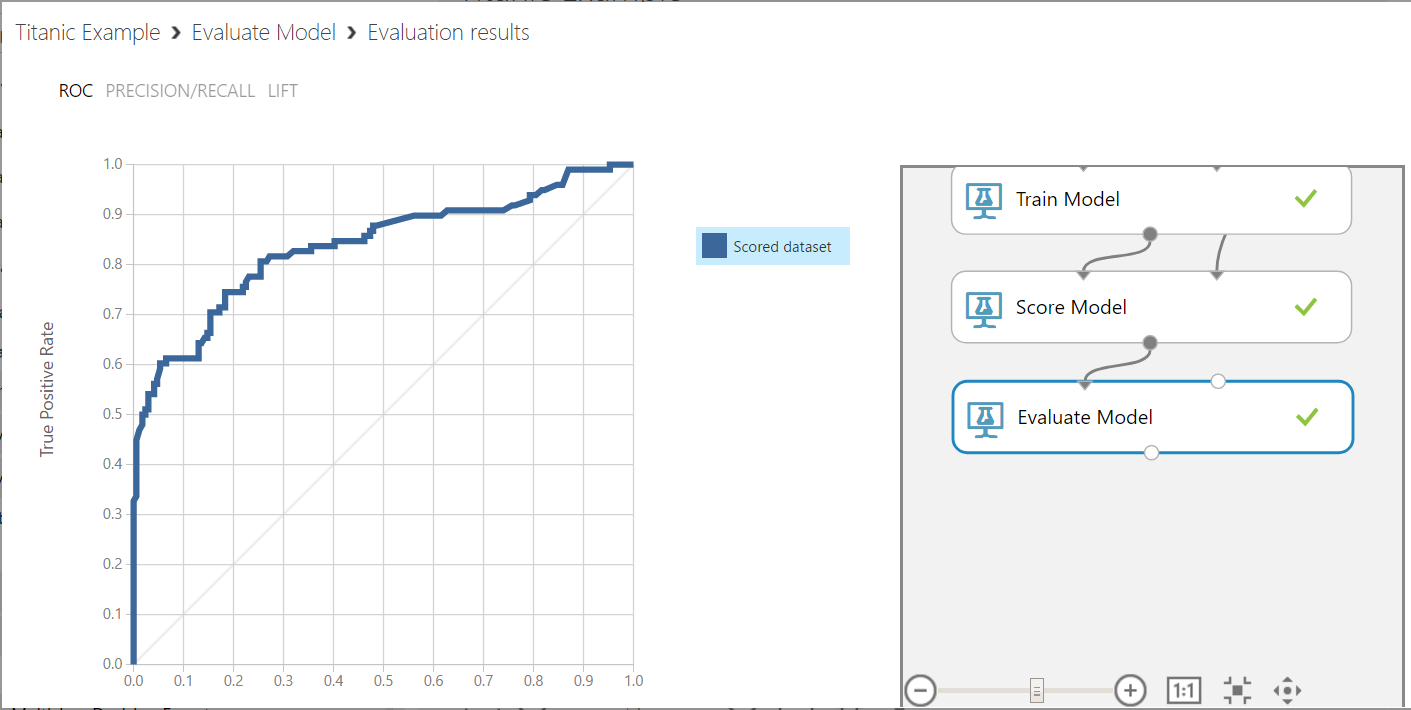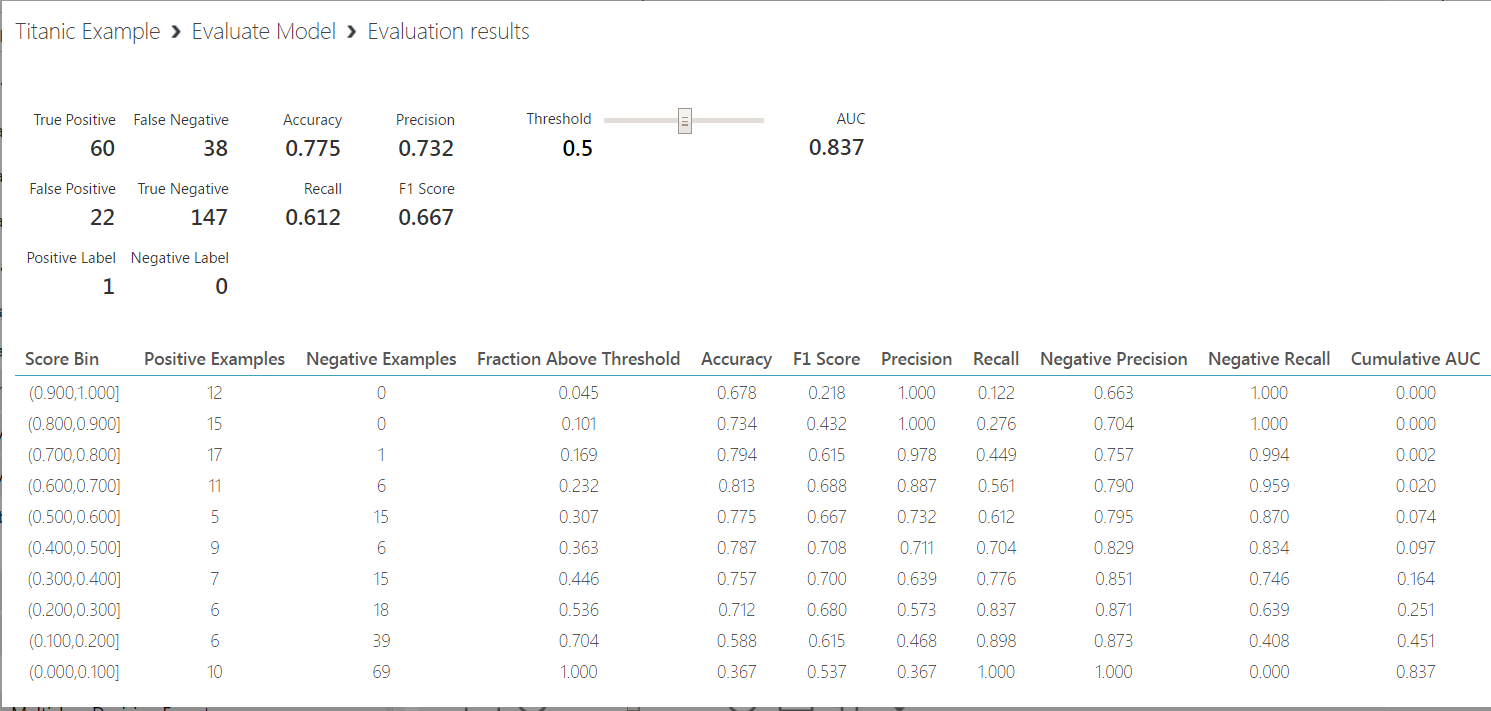From here, we can right click on the Score Model and see the Scored Labels and Probability for given parameters such as Gender, Age etc: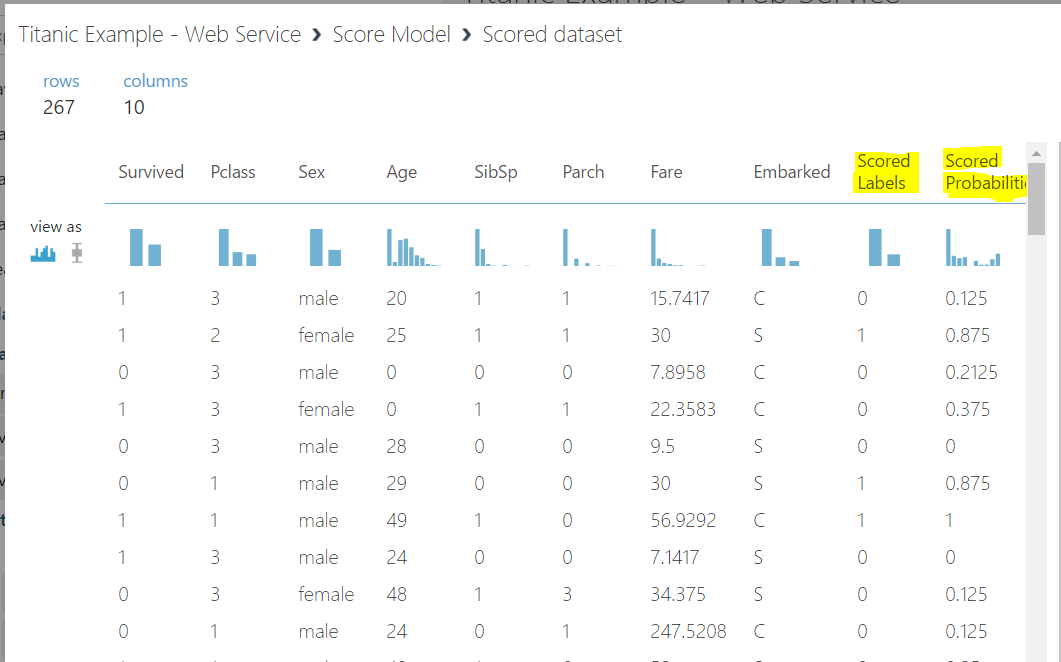In the next post, we will deploy our model in a web service.

I AM SPENDING MORE TIME THESE DAYS CREATING YOUTUBE VIDEOS TO HELP PEOPLE LEARN THE MICROSOFT POWER PLATFORM.

IF YOU WOULD LIKE TO SEE HOW I BUILD APPS, OR FIND SOMETHING USEFUL READING MY BLOG, I WOULD REALLY APPRECIATE YOU SUBSCRIBING TO MY YOUTUBE CHANNEL.

THANK YOU, AND LET'S KEEP LEARNING TOGETHER.

CARL